Author: Oscar Cronquist Article last updated on January 24, 2019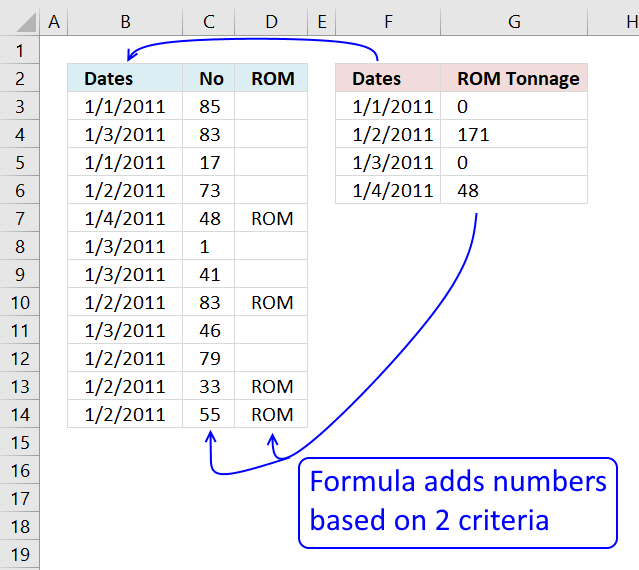I have 57 sheets many of which are linked together by formulas, I need to get numbers from one sheet (A) into another sheet (B).

I need excel to search through the dates in sheet A to find all the data for the date that is selected on sheet B.

then I need it to search in sheet A threw the data for that specific date and select and sum all the data that is catagorised as ROM and put the total in the Cell in Sheet B called ROM Tonnage.

I highly recommend a pivot table for this task if you have lots of data to work with. It is incredibly fast and easy to work with, however, this article demonstrates a formula.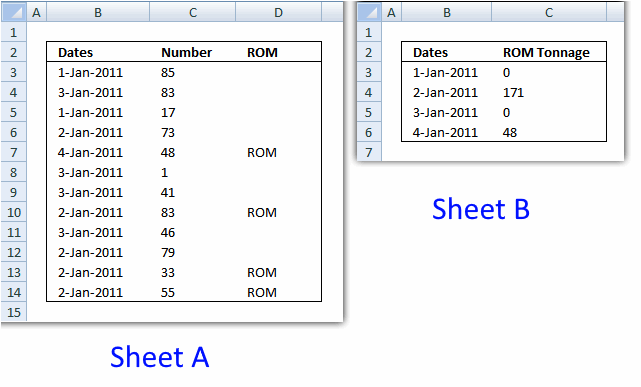Excel 2007 Formula in cell C3, sheet B:

=SUMIFS(A!\$C\$3:\$C\$14, A!\$B\$3:\$B\$14;B!B3, A!\$D\$3:\$D\$14, "ROM")

The SUMIFS function lets you add numbers based on multiple conditions and returns a total, it was introduced in Excel 2007. If you own an earlier version than 2007 then see the formula below.

Excel 2003 Formula in cell C3, sheet B:

=SUMPRODUCT(A!\$C\$3:\$C\$14, --(A!\$B\$3:\$B\$14=B!B3), --(A!\$D\$3:\$D\$14="ROM"))

The SUMPRODUCT function is incredibly useful and easy to use, all conditions are shown in formula above.

### Explaining Excel 2007 formula and later versions

SUMIFS(sum_range, criteria_range1, criteria1,..) adds the cells specified by a given set of conditions or criteria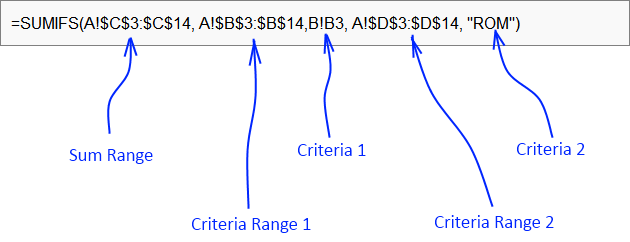### Explaining Excel 2003 formula

#### Step 1 - Cells to sum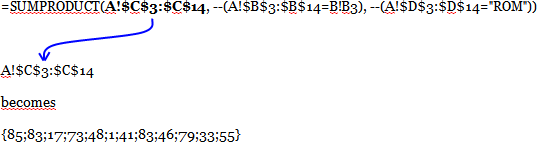#### Step 2 - Find values equal to date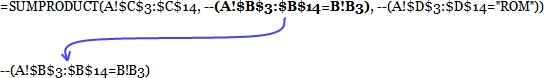becomes

--({40544; 40546; 40544; 40545; 40547; 40546; 40546; 40545; 40546; 40545; 40545; 40545}=40544)

becomes

--({TRUE; FALSE; TRUE; FALSE; FALSE; FALSE; FALSE; FALSE; FALSE; FALSE; FALSE; FALSE})

and returns {1; 0; 1; 0; 0; 0; 0; 0; 0; 0; 0; 0}

#### Step 3 - Find values equal to criterionbecomes

--({0;0;0;0;"ROM";0;0;"ROM";0;0;"ROM";"ROM"}="ROM")

becomes

--({FALSE; FALSE; FALSE; FALSE; TRUE; FALSE; FALSE; TRUE; FALSE; FALSE; TRUE; TRUE})

and returns {0; 0; 0; 0; 1; 0; 0; 1; 0; 0; 1; 1}

#### Step 4 - Putting it all together

SUMPRODUCT(A!\$C\$3:\$C\$14, --(A!\$B\$3:\$B\$14=B!B3), --(A!\$D\$3:\$D\$14="ROM")))

becomes

SUMPRODUCT({85;83; 17;73; 48;1;41; 83;46;79;33;55},{1; 0; 1; 0; 0; 0; 0; 0; 0; 0; 0; 0}, {0; 0; 0; 0; 1; 0; 0; 1; 0; 0; 1; 1})

becomes

SUMPRODUCT({0; 0; 0; 0; 0; 0; 0; 0; 0; 0; 0; 0})

and returns 0 in cell C3.

### Get the Excel filesum-data.xlsx